Search this API

## y.algo Class GraphConnectivity

```java.lang.Objecty.algo.GraphConnectivity
```

`public class GraphConnectivityextends java.lang.Object`

This class provides algorithms for determining certain connectivity components within a graph.

It also provides convenience methods for working with these components.

### Definitions

• Connected graph: A graph is called connected if there exists an undirected path of edges between every pair of nodes.
• Strongly connected graph: A graph is called strongly connected if there exists a directed path between each pair of nodes.
• Biconnected graph: A graph is called biconnected if it has no cut vertex or articulation point (i.e., a node whose removal disconnects the remaining graph).
• KCore: The `k-cores` of a graph are the maximal connected subgraphs in which all nodes have degree greater or equal to `k`.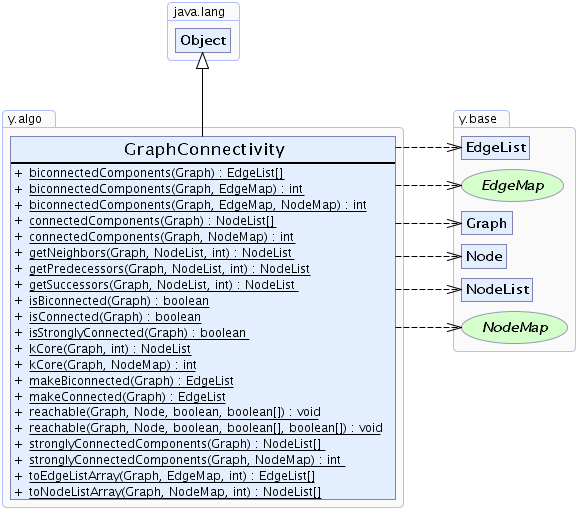Method Summary
`static EdgeList[]` `biconnectedComponents(Graph graph)`
Calculates the biconnected components of a given undirected graph.
`static int` ```biconnectedComponents(Graph graph, EdgeMap compNum)```
Calculates the biconnected components of a given undirected graph and returns their number.
`static int` ```biconnectedComponents(Graph graph, EdgeMap compNum, NodeMap aPoint)```
Calculates the biconnected components and the articulation points of a given undirected graph and returns the number of biconnected components.
`static NodeList[]` `connectedComponents(Graph graph)`
Calculates the connected components of a given graph.
`static int` ```connectedComponents(Graph graph, NodeMap compNum)```
Calculates the connected components of a given graph and returns their number.
`static NodeList` ```getNeighbors(Graph graph, NodeList startNodes, int maxDistance)```
Determines the direct or indirect neighbors of a given set of nodes.
`static NodeList` ```getPredecessors(Graph graph, NodeList startNodes, int maxDistance)```
Determines the direct or indirect predecessors of a given list of nodes.
`static NodeList` ```getSuccessors(Graph graph, NodeList startNodes, int maxDistance)```
Determines the direct or indirect successors of a given list of nodes.
`static boolean` `isBiconnected(Graph graph)`
Checks whether or not the given undirected graph is biconnected.
`static boolean` `isConnected(Graph graph)`
Checks whether or not the given graph is connected.
`static boolean` `isStronglyConnected(Graph graph)`
Checks whether or not the given directed graph is strongly connected.
`static NodeList` ```kCore(Graph graph, int k)```
Calculates the k-core for a given undirected input graph and k value.
`static int` ```kCore(Graph graph, NodeMap kValue)```
Calculates the k-cores of an undirected input graph.
`static EdgeList` `makeBiconnected(Graph graph)`
Makes the given graph biconnected by inserting a minimum number of edges in the graph.
`static EdgeList` `makeConnected(Graph graph)`
Makes a graph connected by adding additional edges to the graph.
`static void` ```reachable(Graph graph, Node start, boolean directed, boolean[] reached)```
Determines the set of nodes that are reachable from a given node.
`static void` ```reachable(Graph graph, Node start, boolean directed, boolean[] forbidden, boolean[] reached)```
Determines the set of nodes that are reachable from a given node when a set of edges that cannot be traversed is specified.
`static NodeList[]` `stronglyConnectedComponents(Graph graph)`
Calculates the strongly connected components of a given graph.
`static int` ```stronglyConnectedComponents(Graph graph, NodeMap compNum)```
Calculates the strongly connected components of a given graph and returns their number.
`static EdgeList[]` ```toEdgeListArray(Graph graph, EdgeMap compNum, int maxCompNum)```
Transforms the return values of `biconnectedComponents(Graph, EdgeMap)` to an array of `EdgeList`s, like it is returned by `biconnectedComponents(Graph)`.
`static NodeList[]` ```toNodeListArray(Graph graph, NodeMap compNum, int maxCompNum)```
Transforms the return values of method `connectedComponents(Graph, NodeMap)` to an array of `NodeList`s, like it is returned by `connectedComponents(Graph)`.

Methods inherited from class java.lang.Object
`clone, equals, finalize, getClass, hashCode, notify, notifyAll, toString, wait, wait, wait`

Method Detail

### connectedComponents

`public static NodeList[] connectedComponents(Graph graph)`
Calculates the connected components of a given graph.

A graph `G` is called connected if there exists an undirected path of edges between every pair of nodes.

The connected components of a graph are the maximal connected subgraphs of which the graph consists.

Complexity:
`O(graph.N() + graph.E())`
Parameters:
`graph` - the input graph
Returns:
an array of `NodeList`s each of which contains the nodes that belong to the same connected component

### connectedComponents

```public static int connectedComponents(Graph graph,
NodeMap compNum)```
Calculates the connected components of a given graph and returns their number.

A graph `G` is called connected if there exists an undirected path of edges between every pair of nodes.

The connected components of a graph are the maximal connected subgraphs of which the graph consists.

Complexity:
`O(graph.N() + graph.E())`
Parameters:
`compNum` - the `NodeMap` that will be filled during the execution and returns the zero-based index of the connected component to which each node belongs
Returns:
the number of connected components of the given graph

### makeConnected

`public static EdgeList makeConnected(Graph graph)`
Makes a graph connected by adding additional edges to the graph.

The number of edges that will be added equals the number of separate components of the original graph minus `1`.

Complexity:
`O(graph.N() + graph.E())`
Parameters:
`graph` - the input graph
Returns:
an `EdgeList` containing the edges added to the graph

### toNodeListArray

```public static NodeList[] toNodeListArray(Graph graph,
NodeMap compNum,
int maxCompNum)```
Transforms the return values of method `connectedComponents(Graph, NodeMap)` to an array of `NodeList`s, like it is returned by `connectedComponents(Graph)`.

Parameters:
`graph` - the input graph
`compNum` - the `NodeMap` that will be filled during the execution and returns the zero-based index of the connected component to which each node belongs
`maxCompNum` - the maximum number of connected components
Returns:
an array of `NodeList`s each of which contains the nodes that belong to the same connected component

### isConnected

`public static boolean isConnected(Graph graph)`
Checks whether or not the given graph is connected.

A graph is called connected if there exists an undirected path of edges between every pair of nodes.

Parameters:
`graph` - the given graph
Returns:
`true` if the graph is connected, `false` otherwise
Sample Graphs: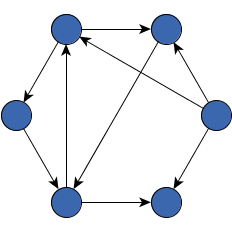Connected graphNon-connected graph

### biconnectedComponents

`public static EdgeList[] biconnectedComponents(Graph graph)`
Calculates the biconnected components of a given undirected graph.

The result is returned as an array of `EdgeList`s each containing all edges that belong to the same biconnected component.

Self-loops do not belong to any biconnected component. Therefore, no self-loops are included in the returned `EdgeList`s.
Precondition:
The graph must be `connected`.
Complexity:
`O(graph.N() + graph.E())`
Parameters:
`graph` - the input graph
Returns:
an array of `EdgeList`s each containing all edges that belong to the same biconnected component
`biconnectedComponents(Graph, EdgeMap)`, `biconnectedComponents(Graph, EdgeMap, NodeMap)`

### biconnectedComponents

```public static int biconnectedComponents(Graph graph,
EdgeMap compNum)```
Calculates the biconnected components of a given undirected graph and returns their number.

The main result is returned in the form of an `EdgeMap` that provides for each edge the zero-based index of the biconnected component to which it belongs.

Self-loops do not belong to any biconnected component. Therefore, the component index for self-loops is always `-1`.
Preconditions:
The graph must be `connected`.
`compNum` must not be `null`.
Complexity:
`O(graph.N() + graph.E())`
Parameters:
`graph` - the input graph
`compNum` - the `EdgeMap` that will be filled during the execution and returns the zero-based index of the biconnected component to which each edge belongs or `-1` for self-loops
Returns:
the number of biconnected components
`biconnectedComponents(Graph)`, `biconnectedComponents(Graph, EdgeMap, NodeMap)`

### biconnectedComponents

```public static int biconnectedComponents(Graph graph,
EdgeMap compNum,
NodeMap aPoint)```
Calculates the biconnected components and the articulation points of a given undirected graph and returns the number of biconnected components.

Articulation points are returned in the form of a `NodeMap` that returns for each node a boolean value indicating whether or not it is an articulation point.

Self-loops do not belong to any biconnected component. Therefore, the component index for self-loops is always `-1`.
Preconditions:
The graph must be `connected`.
`compNum` should not be `null`.
`aPoint` should not be `null`.
Complexity:
`O(graph.N() + graph.E())`
Parameters:
`graph` - the input graph
`compNum` - the `EdgeMap` that will be filled during the execution and returns the zero-based index of the biconnected component to which each edge belongs or `-1` for self-loops
`aPoint` - the `NodeMap` that will be filled during the execution and returns a boolean value indicating whether or not a given node is an articulation point
Returns:
the number of biconnected components
`biconnectedComponents(Graph)`, `biconnectedComponents(Graph, EdgeMap)`

### toEdgeListArray

```public static EdgeList[] toEdgeListArray(Graph graph,
EdgeMap compNum,
int maxCompNum)```
Transforms the return values of `biconnectedComponents(Graph, EdgeMap)` to an array of `EdgeList`s, like it is returned by `biconnectedComponents(Graph)`.

Parameters:
`graph` - the input graph
`compNum` - the `EdgeMap` that will be filled during the execution and returns the zero-based index of the connected component to which each edge belongs
`maxCompNum` - the maximum number of biconnected components
Returns:
an array of `EdgeList`s each containing all edges that belong to the same biconnected component

### makeBiconnected

`public static EdgeList makeBiconnected(Graph graph)`
Makes the given graph biconnected by inserting a minimum number of edges in the graph.

The given graph is considered to be undirected.

Precondition:
The graph must be `connected`.
Complexity:
`O(graph.N() + graph.E())`
Parameters:
`graph` - the input graph
Returns:
an `EdgeList` containing the edges added to the graph

### isBiconnected

`public static boolean isBiconnected(Graph graph)`
Checks whether or not the given undirected graph is biconnected.

A graph is called biconnected if it has no cut vertex or articulation point, i.e., no node whose removal disconnects the graph.

Parameters:
`graph` - the given undirected graph
Returns:
`true` if the graph is biconnected, `false` otherwise
Sample Graphs: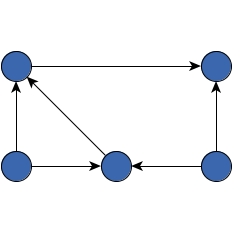Biconnected graphNon-biconnected graph

### reachable

```public static void reachable(Graph graph,
Node start,
boolean directed,
boolean[] reached)```
Determines the set of nodes that are reachable from a given node.

The result is based on a depth first search.

Precondition:
`reached.length = graph.N()`
Parameters:
`graph` - the given graph
`start` - the node from which the search starts
`directed` - `true` if the edges should be traversed from source to target, `false` if edges can be traversed in both directions
`reached` - an array that will be filled during the execution and returns for each `Node` a `Boolean` value based on whether the node can be reached during the DFS; if a node `v` is reachable, then `reached[v.index()] = true`

### reachable

```public static void reachable(Graph graph,
Node start,
boolean directed,
boolean[] forbidden,
boolean[] reached)```
Determines the set of nodes that are reachable from a given node when a set of edges that cannot be traversed is specified.

The result is based on a depth first search.

Precondition:
`forbiddenEdges.length = graph.E()`
Parameters:
`graph` - the given graph
`start` - the node from which the search starts
`directed` - `true` if the edges should be traversed from source to target, `false` if edges can be traversed in both directions
`forbidden` - an array that holds for each `Edge` a `Boolean` value indicating whether or not an edge can be traversed; an edge `e` is marked as forbidden if `forbidden[e.index()] == true`
`reached` - an array that will be filled during the execution and returns for each `Node` a `Boolean` value based on whether the node can be reached during the DFS; if a node `v` is reachable, then `reached[v.index()] = true`

### getSuccessors

```public static NodeList getSuccessors(Graph graph,
NodeList startNodes,
int maxDistance)```
Determines the direct or indirect successors of a given list of nodes.

• A direct successor of a node is the target node of an outgoing edge connected to a node.
• An indirect successor of a node is a direct successor to another successor of a node.

The order of the returned nodes is determined by a breadth first search. No start node will be part of the resulting set.

To obtain the result, an integer value should be given as input that limits the distance between a start node and a returned node. For all returned nodes there must be a path to a start node that has a length equal to or smaller than this distance.

Setting the maximum distance to `1` will only yield the direct successors of all start nodes. On the other hand, setting the maximum distance to `graph.N()` or larger will yield all successors of all start nodes.

Parameters:
`graph` - the given graph
`startNodes` - a `NodeList` containing the nodes from which the search starts
`maxDistance` - an integer value that limits the distance between a start node and a returned node
Returns:
a `NodeList` that contains all direct and indirect successors of a node

### getPredecessors

```public static NodeList getPredecessors(Graph graph,
NodeList startNodes,
int maxDistance)```
Determines the direct or indirect predecessors of a given list of nodes.

• A direct predecessor of a node is the source node of an incoming edge connected to a node.
• An indirect predecessor of a node is a direct predecessor to another predecessor of a node.

The order of the returned nodes is determined by a breadth first search. No start node will be part of the resulting set.

To obtain the result, an integer value should be given as input that limits the distance between a start node and a returned node. For all returned nodes there must be a path to a start node that has a length equal to or smaller than this distance.

Setting the maximum distance to `1` will only yield the direct predecessors of all start nodes. On the other hand, setting the maximum distance to `graph.N()` or larger will yield all predecessors of all start nodes.

Parameters:
`graph` - the given graph
`startNodes` - a `NodeList` containing the nodes from which the search starts
`maxDistance` - an integer value that limits the distance between a start node and a returned node
Returns:
a `NodeList` that contains all direct and indirect predecessors of a node

### getNeighbors

```public static NodeList getNeighbors(Graph graph,
NodeList startNodes,
int maxDistance)```
Determines the direct or indirect neighbors of a given set of nodes.

• A direct neighbor of a node is directly connected by an edge to that node.
• An indirect neighbor of a node is directly connected to another direct or indirect neighbor of a node.

The order of the returned nodes is determined by a breadth first search. No start node will be part of the resulting set.

To obtain the result, an integer value should be given as input that limits the distance between a start node and a returned node. For all returned nodes there must be a path to a start node that has a length equal to or smaller than this distance.

Setting the maximum distance to `1` will only yield the direct neighbors of all start nodes. On the other hand, setting the maximum distance to `graph.N()` or larger will yield all neighbors of all start nodes.

Returns:
a `NodeList` that contains all direct and indirect neighbors of a node

### stronglyConnectedComponents

`public static NodeList[] stronglyConnectedComponents(Graph graph)`
Calculates the strongly connected components of a given graph.

A graph is called strongly connected if there exists a directed path between each pair of nodes.

The strongly connected components of a graph are the strongly connected subgraphs of which it consists.

Complexity:
`O(graph.N() + graph.E())`
Parameters:
`graph` - the input graph
Returns:
an array of `NodeList`s each of which contains the nodes that belong to the same strongly connected component
`stronglyConnectedComponents(Graph, NodeMap)`

### stronglyConnectedComponents

```public static int stronglyConnectedComponents(Graph graph,
NodeMap compNum)```
Calculates the strongly connected components of a given graph and returns their number.

A graph is called strongly connected if there exists a directed path between each pair of nodes.

The strongly connected components of a graph are the strongly connected subgraphs of which it consists.

Complexity:
`O(graph.N() + graph.E())`
Parameters:
`graph` - the input graph
`compNum` - the `NodeMap` that will be filled during the execution and returns the zero-based index of the connected component to which each node belongs
Returns:
the number of strongly connected components of the given graph
`stronglyConnectedComponents(Graph)`

### isStronglyConnected

`public static boolean isStronglyConnected(Graph graph)`
Checks whether or not the given directed graph is strongly connected.

A graph is called strongly connected if there exists a directed path between each pair of nodes.

Parameters:
`graph` - the given directed graph
Returns:
`true` if the graph is strongly connected, `false`, otherwise
Sample Graphs: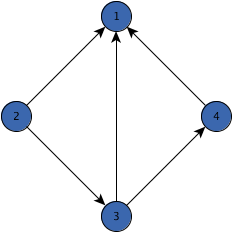Strongly connected graph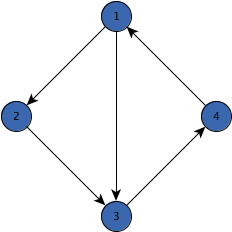Non-strongly connected graph

### kCore

```public static int kCore(Graph graph,
NodeMap kValue)```
Calculates the k-cores of an undirected input graph.

The k-core of an undirected input graph consists of the subgraph components where each node has at least degree `k`. The algorithm has runtime complexity `O((n+m)log(n))` and requires linear space.

For a given `k`, the k-core of a graph can consists of all nodes with `kValue` value greater or equal to `k`.
Parameters:
`graph` - the undirected input graph
`kValue` - stores the largest `k` for which a node is contained in the k-core
Returns:
the largest `k` for which the k-core of the graph is not empty

### kCore

```public static NodeList kCore(Graph graph,
int k)```
Calculates the k-core for a given undirected input graph and k value.

The k-core of an undirected input graph consists of the subgraph components where each node has at least degree `k`. The algorithm has runtime complexity `O((n+m)log(n))` and requires linear space.

This algorithm doesn't modify the input graph but returns the nodes of the k-core (list may be empty).
Parameters:
`graph` - the undirected input graph
`k` - the minimum degree (k-core) of a node in the resulting subgraph components
Returns:
a list that contains the nodes of the k-core (list may be empty)